﻿ 基于空间矢量算法的三电平逆变器的研究

# 基于空间矢量算法的三电平逆变器的研究Research on Three-Level Inverter Based on Space Vector Algorithm

Abstract: This paper designs a structure of high power magnetron drive power, inverter module using a three-level inverter as a magnetron anode drive circuit. A mathematical model of the magnetron anode drive circuit was established. After mathematical analysis, the output voltage of the magnetron anode drive circuit under different switching states is derived. A simulation model was established in MATLAB, which uses SPWM and SVPWM to drive the three-level inverter. Through the comparison of simulation data, the superiority of space vector algorithm is verified from three aspects. At the same time, the waveform of the output voltage and current is obtained, which provides a theoretical basis for the subsequent application of this structure to the microwave generating device.

1. 引言

2. 磁控管驱动电源的拓扑结构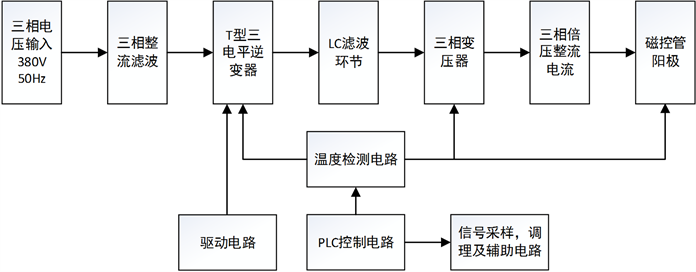Figure 1. Anode circuit flow chart using a three-level inverter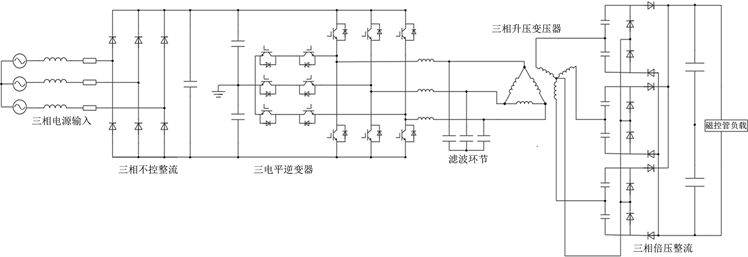Figure 2. Anode circuit diagram using a three-level inverter

3. 三电平逆变器的数学模型和矢量控制

3.1. 三电平逆变器的数学模型

${U}_{io}=\frac{{S}_{i}}{2}{U}_{dc}$ (1)

 (2)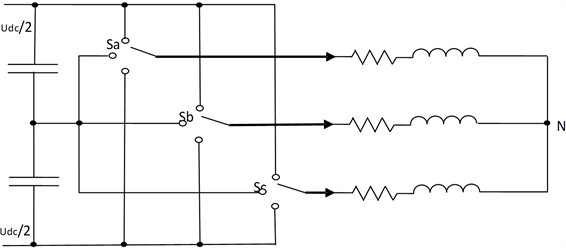Figure 3. Three-level inverter simplified model

${U}_{AN}+{U}_{BN}+{U}_{CN}=0$ (3)

$\left[\begin{array}{c}{U}_{AN}\\ {U}_{BN}\\ {U}_{CN}\end{array}\right]=\frac{1}{3}\left[\begin{array}{ccc}1& 0& -1\\ -1& 1& 0\\ 0& -1& 1\end{array}\right]\left[\begin{array}{c}{U}_{AB}\\ {U}_{BC}\\ {U}_{CA}\end{array}\right]$ (4)

${S}_{a},\text{\hspace{0.17em}}{S}_{b},\text{\hspace{0.17em}}{S}_{c}$ 表示输出线电压，则(5)式可以表示为：

$\left[\begin{array}{c}{U}_{AN}\\ {U}_{BN}\\ {U}_{CN}\end{array}\right]=\frac{{U}_{dc}}{6}\left[\begin{array}{ccc}2& -1& -1\\ -1& 2& -1\\ -1& -1& 2\end{array}\right]\left[\begin{array}{c}{S}_{a}\\ {S}_{b}\\ {S}_{c}\end{array}\right]$ (5)

$\begin{array}{c}\stackrel{\to }{U}={U}_{\alpha }+j{U}_{\beta }\\ ={U}_{A}+j\left(\frac{1}{\sqrt{3}}{U}_{B}-\frac{1}{\sqrt{3}}{U}_{C}\right)\\ =\left(\frac{2}{3}{U}_{A}-\frac{1}{3}{U}_{B}-\frac{1}{3}{U}_{C}\right)+j\left(\frac{1}{\sqrt{3}}{U}_{B}-\frac{1}{\sqrt{3}}{U}_{C}\right)\\ =\frac{2}{3}\left[{U}_{A}+\left(-\frac{1}{2}+j\frac{\sqrt{3}}{2}\right){U}_{B}+\left(-\frac{1}{2}-j\frac{\sqrt{3}}{2}\right){U}_{C}\right]\\ =\frac{2}{3}\left({U}_{A}+{e}^{j\frac{2}{3}\pi }{U}_{B}+{e}^{-j\frac{2}{3}\pi }{U}_{C}\right)\end{array}$ (6)

 (7)

${S}_{a},\text{\hspace{0.17em}}{S}_{b},\text{\hspace{0.17em}}{S}_{c}$ 的27种开关状态带入(7)式中，在αβ坐标系下画出矢量分布，如图4所示。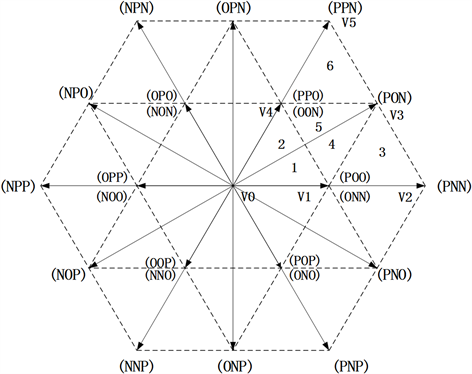Figure 4. Space vector distribution

3.2. 空间矢量调制的优势

1) 直流电压利用率高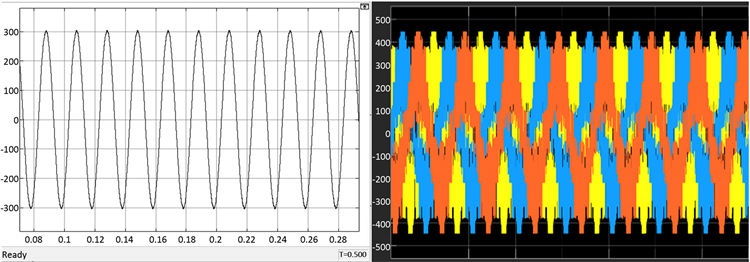Figure 5. Output line voltage waveform

2) 总谐波畸变率小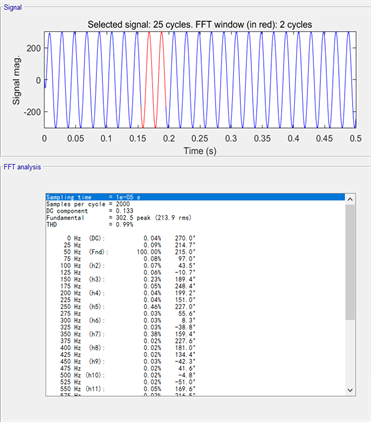Figure 6. Total Harmonics Distortion

3) 可以平衡中点电压

3.3. 空间矢量算法在三电平逆变器中的实现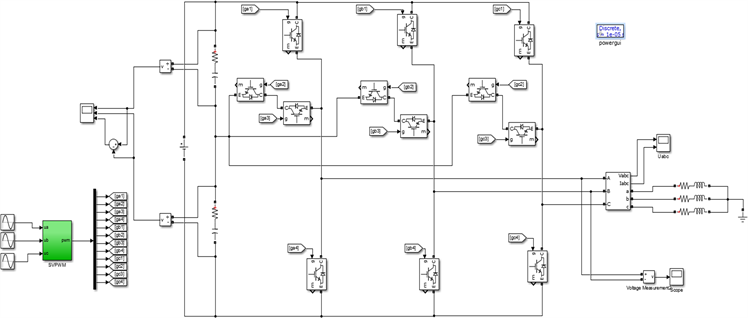Figure 7. Three-level inverter simulation model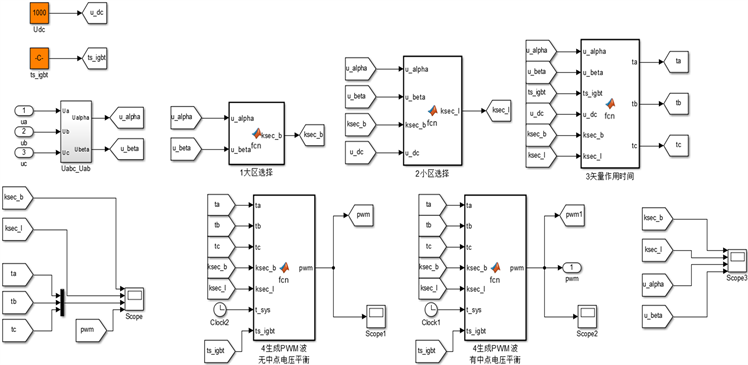Figure 8. Vector control module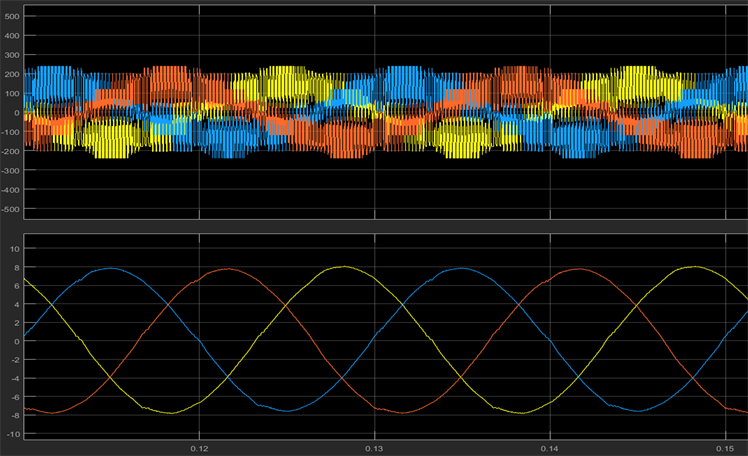Figure 9. Output voltage and current waveform

4. 结语

 邓永峰, 印长豹, 刘凯. 微波源用大功率高压直流电源[J]. 真空电子技术, 2016(2): 58-61.

 Schweizer, M. and Kolar, J.W. (2013) Design and Implementation of a Highly Efficient Three-Level T-Type Converter for Low-Voltage Applications. IEEE Transactions on Power Electronics, 28, 899-907.
https://doi.org/10.1109/TPEL.2012.2203151

 才琳, 孙耀杰, 高骞, 等. 磁控管电源系统研究与设计[J]. 电子设计工程, 2011, 19(23): 154-155.

 Pietta, L.P., Treter, M.E., Barin, J.S. and Michels, L. (2015) Modelling and Control of a High-Frequency Magnetron Power Supply for Microwave Heating Applications. 2015 IEEE 13th Brazilian Power Electronics Conference and 1st Southern Power Electronics Conference (COBEP/SPEC), Fortaleza, 29 November-2 December 2015, 1-6.
https://doi.org/10.1109/COBEP.2015.7420098

 陈家新, 汤勇, 沈勇, 等. 微波加热用磁控管电源的设计[J]. 电子设计工程, 2015(24): 80-83.

 张兆镗. 磁控管与微波加热技术[M]. 成都: 电子科技大学出版社, 2017.

 Heggannavar, M. and Kulkarni, H. (2015) Design of Magnetron Power Source from Three Phase Supply. 2015 International Conference on Energy Systems and Applications, Pune, 30 October-1 November 2015, 552-556.
https://doi.org/10.1109/ICESA.2015.7503410

 袁庆庆, 伍小杰, 吴强. 低开关频率下的不对称空间矢量脉宽调制[J]. 电力电子技术, 2013, 47(5): 4-6.

 李亚维, 邓建军, 谢敏, 等. 800 kV/100 Hz高功率微波驱动电源控制系统[J]. 高电压技术, 2009(6): 1426-1429.

 王强, 刘岩松, 陈祥雪, 等. 具有对称辅助电路的谐振直流环节三电平软开关逆变器[J]. 中国电机工程学报, 2014, 34(27): 4584-4593.

Top